Next: Projectile motion Up: Motion in 3 dimensions Previous: Motion with constant velocity

## Motion with constant acceleration

An object moving in 3 dimensions with constant acceleration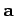possesses a vector displacement of the form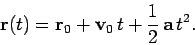(62)

Hence, the object's velocity is given by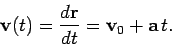(63)

Note that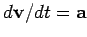, as expected. In the above, the constant vectors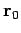and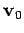are the object's displacement and velocity at time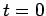, respectively.

As is easily demonstrated, the vector equivalents of Eqs. (21)-(23) are: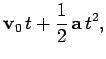(64)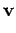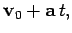(65)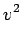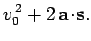(66)

These equation fully characterize 3-dimensional motion with constant acceleration. Here,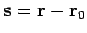is the net displacement of the object between timesand.

The quantity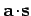, appearing in Eq. (66), is termed the scalar product of vectorsand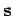, and is defined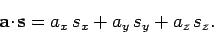(67)

The above formula has a simple geometric interpretation, which is illustrated in Fig. 15. If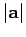is the magnitude (or length) of vector,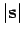is the magnitude of vector, andis the angle subtended between these two vectors, then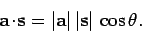(68)

In other words, the scalar product of vectorsandequals the product of the length of vectortimes the length of that component of vectorwhich lies in the same direction as vector. It immediately follows that if two vectors are mutually perpendicular (i.e.,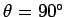) then their scalar product is zero. Furthermore, the scalar product of a vector with itself is simply the magnitude squared of that vector [this is immediately apparent from Eq. (67)]: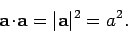(69)

It is also apparent from Eq. (67) that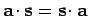, and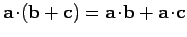, and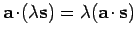.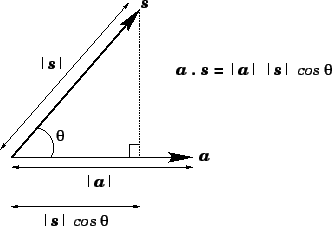Incidentally, Eq. (66) is obtained by taking the scalar product of Eq. (65) with itself, taking the scalar product of Eq. (64) with, and then eliminating.Next: Projectile motion Up: Motion in 3 dimensions Previous: Motion with constant velocity
Richard Fitzpatrick 2006-02-02